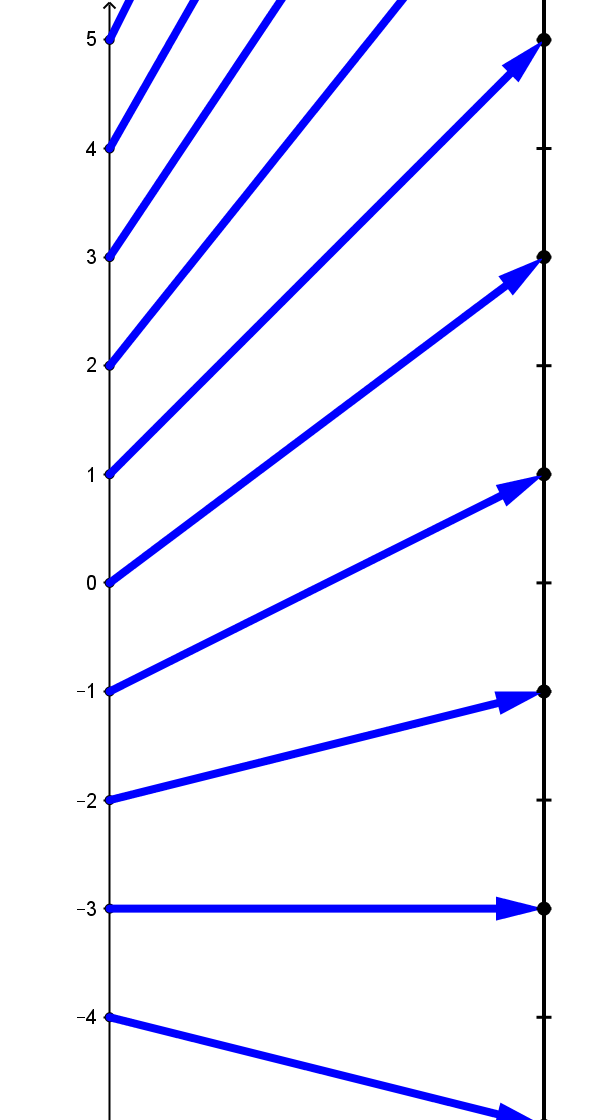### SectionINLinear Functions

Linear Functions

Introduction: Of all the functions that are studied, the simplest and most common class of functions is the class of linear functions. These functions are characterized by the simplest of operations: multiplication by a constant and addition of a constant.

For  example, the function defined by assigning the value $f(x) = 2x+3$ to the number $x$, also represented symbolically as $f: x \rightarrow 2x+3$  ,  or verbally  by saying "to find $f(x)$, multiply $x$ by 2 then add 3", is described as a linear function. This  function was visualized in Chapter 1, VF,  by the  mapping diagram, TMD1.Mapping Diagram TMD1

This section will provide examples, explanations, exercises and problems that will help students use the power of the mapping diagram  along with the three other tools (equations, tables , and graphs) to understand linear functions.

#### Linear Function Definition

##### LF.LFILinear Functions are Important.
Example LF.0 The First Linear Function Example.
This example presents the linear function $f(x) = 2x + 3$ with a table of data,  a graph and a mapping diagram. The "linear coefficient" is $2$ and the "constant " is $3$.

Treatment of linear functions and their graphical interpretation with lines and equations are familiar. [See wikipedia.org/wiki : Linear_function_(calculus)]
They appear in every textbook that deals at all with beginning algebra and coordinate geometry- from algebra I to beginning calculus. What is missing is a balanced treatment using mapping diagrams to reinforce the function aspect of visualization.  That will be emphasis of this section.

Comparisons will be made when appropriate to graphs- but we will develop the basic concepts for linear functions with mapping diagrams. The end of this section includes some powerful and different ways to think about linear functions and the ways they are represented algebraically.

Here is a YouTube video (about 10 minutes) that covers some initial work on using mapping diagrams to visualize linear functions:

##### Subsection LF.APPLinear Function Applications (not yet ready)

Here is a link to a spreadsheet for exploring the effects of $m$ and $b$ on the mapping diagram for a linear function.